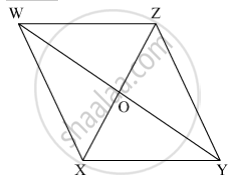Share

# Balbharati solutions for Maharashtra state board Class 8 Mathematics chapter 8 - Quadrilateral : Constructions and Types [Latest edition]

Textbook page

#### Chapters## Chapter 8: Quadrilateral : Constructions and Types

Practice Set 8.1Practice Set 8.2Practice Set 8.3

#### Balbharati solutions for Maharashtra state board Class 8 Mathematics Chapter 8 Quadrilateral : Constructions and Types Exercise Practice Set 8.1 [Page 43]

Practice Set 8.1 | Q 1 | Page 43

Construct the following quadrilateral of given measures.

In ☐ MORE, l(MO) = 5.8 cm, l(OR) = 4.4 cm, m∠M = 58°, m∠O = 105°, m∠R = 90° .

Practice Set 8.1 | Q 2 | Page 43

Construct the following quadrilateral of given measures.

Construct ☐ DEFG such that l(DE) = 4.5 cm, l(EF) = 6.5 cm, l(DG) = 5.5 cm, l(DF) = 7.2 cm, l(EG) = 7.8 cm.

Practice Set 8.1 | Q 3 | Page 43

Construct the following quadrilateral of given measures.

In ☐ ABCD l(AB) = 6.4 cm, l(BC) = 4.8 cm, m∠A = 70° , m∠B = 50° , m∠C = 140°.

Practice Set 8.1 | Q 4 | Page 43

Construct the following quadrilateral of given measures.

Construct ☐ LMNO such that l(LM) = l(LO) = 6 cm, l(ON) = l(NM) = 4.5 cm, l(OM) = 7.5 cm.

#### Balbharati solutions for Maharashtra state board Class 8 Mathematics Chapter 8 Quadrilateral : Constructions and Types Exercise Practice Set 8.2 [Pages 46 - 47]

Practice Set 8.2 | Q 1 | Page 46

Draw a rectangle ABCD such that l(AB) = 6.0 cm and l (BC) = 4.5 cm.

Practice Set 8.2 | Q 2 | Page 46

Draw a square WXYZ with side 5.2 cm.

Practice Set 8.2 | Q 3 | Page 46

Draw a rhombus KLMN such that its side is 4 cm and m∠K = 75° .

Practice Set 8.2 | Q 4 | Page 46

If diagonal of a rectangle is 26 cm and one side is 24 cm, find the other side.

Practice Set 8.2 | Q 5 | Page 47

Lengths of diagonals of a rhombus ABCD are 16 cm and 12 cm. Find the side and perimeter of the rhombus.

Practice Set 8.2 | Q 6 | Page 47

Find the length of diagonal of a square with side 8 cm .

Practice Set 8.2 | Q 7 | Page 47

Measure of one angle of a rhombus is 50° .find the measures of remaining three angles.

#### Balbharati solutions for Maharashtra state board Class 8 Mathematics Chapter 8 Quadrilateral : Constructions and Types Exercise Practice Set 8.3 [Pages 49 - 50]

Practice Set 8.3 | Q 1 | Page 49

Measures of opposite angles of a parallelogram are (3x − 2)° and (50 − x)° . Find the measure of its each angle.

Practice Set 8.3 | Q 2 | Page 50

Referring the adjacent figure of a parallelogram, write the answer of questions given below.(1) If l(WZ) = 4.5 cm then l(XY) = ?
(2) If l(YZ) = 8.2 cm then l(XW) = ?
(3) If l(OX) = 2.5 cm then l(OZ) = ?
(4) If l(WO) = 3.3 cm then l(WY) = ?
(5) If m∠WZY = 120° then m∠WXY = ? and m∠XWZ = ?

Practice Set 8.3 | Q 3 | Page 50

Construct a parallelogram ABCD such that l(BC) = 7 cm, m∠ABC = 40° , l(AB) = 3 cm.

Practice Set 8.3 | Q 4 | Page 50

Ratio of consecutive angles of a quadrilateral is 1:2:3:4. Find the measure of its each angle. Write, with reason, what type of a quadrilateral it is.

Practice Set 8.3 | Q 5 | Page 50

Construct ☐ BARC such that l(BA) = l(BC) = 4.2 cm, l(AC) = 6.0 cm, l(AR) = l(CR) = 5.6 cm

Practice Set 8.3 | Q 6 | Page 50

Construct ☐ PQRS, such that l(PQ) = 3.5 cm, l(QR) = 5.6 cm, l(RS) = 3.5 cm, m∠Q = 110° , m∠R = 70° . If it is given that ☐ PQRS is a parallelogram, which of the given information is unnecessary?

## Chapter 8: Quadrilateral : Constructions and Types

Practice Set 8.1Practice Set 8.2Practice Set 8.3## Balbharati solutions for Maharashtra state board Class 8 Mathematics chapter 8 - Quadrilateral : Constructions and Types

Balbharati solutions for Maharashtra state board Class 8 Mathematics chapter 8 (Quadrilateral : Constructions and Types) include all questions with solution and detail explanation. This will clear students doubts about any question and improve application skills while preparing for board exams. The detailed, step-by-step solutions will help you understand the concepts better and clear your confusions, if any. Shaalaa.com has the Maharashtra State Board Maharashtra state board Class 8 Mathematics solutions in a manner that help students grasp basic concepts better and faster.

Further, we at Shaalaa.com provide such solutions so that students can prepare for written exams. Balbharati textbook solutions can be a core help for self-study and acts as a perfect self-help guidance for students.

Concepts covered in Maharashtra state board Class 8 Mathematics chapter 8 Quadrilateral : Constructions and Types are Construction of a Quadrilateral, Concept of a Rectangle, Concept of a Square, Concept of Rhombus, Concept of Parallelogram, Concept of Trapezium, Concept of Kite.

Using Balbharati Class 8 solutions Quadrilateral : Constructions and Types exercise by students are an easy way to prepare for the exams, as they involve solutions arranged chapter-wise also page wise. The questions involved in Balbharati Solutions are important questions that can be asked in the final exam. Maximum students of Maharashtra State Board Class 8 prefer Balbharati Textbook Solutions to score more in exam.

Get the free view of chapter 8 Quadrilateral : Constructions and Types Class 8 extra questions for Maharashtra state board Class 8 Mathematics and can use Shaalaa.com to keep it handy for your exam preparation